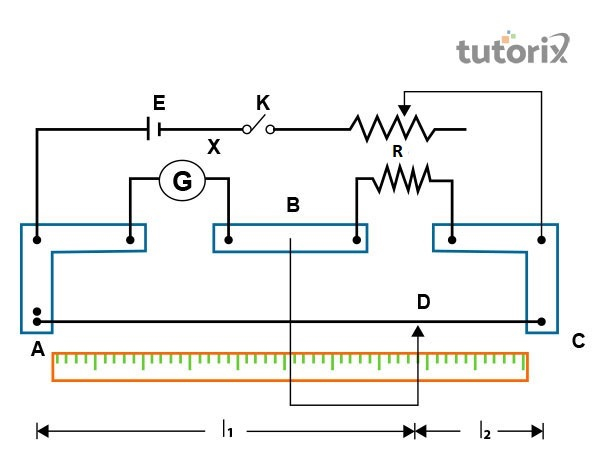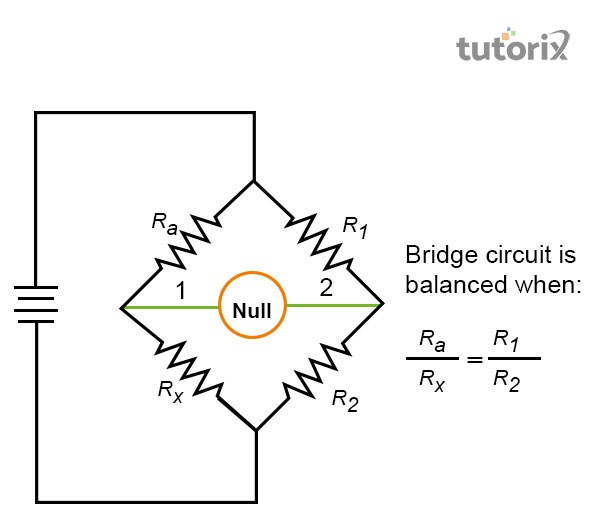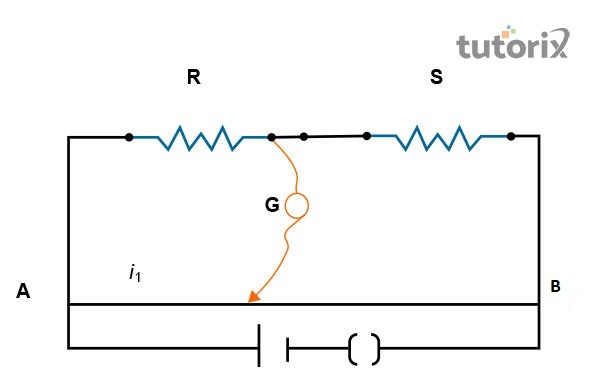# To find the Resistance of given wire using Metre Bridge and hence determine the Resistivity of its Material Experiment

## Introduction

In order to determine the resistivity power of the material, the metre bridge is accepted for the utilisation with the different materials. Different materials need to be utilised to operate the experiment successfully with some precautions to avoid any unwanted errors. In this tutorial, the resistivity power of a material is highlighted with the utilisation of a metre bridge. Moreover, this study aims to determine the specific resistance of any given material with the application of the metre bridge.

A metre bridge or the slide wire bridge is an apparatus that operations based on the principle of the Wheatstone bridge. In most use cases, the resistance of a wire is measured with the principle of the Wheatstone bridge and the successful experiment resolves the usefulness of the application of the metre bridge. A metre bridge includes a wire with a length of 1 m and it is stretching the areas by reforming the cross-sectional direction. In this instrument, the endpoints of the used wires are connected with a cell which is also clamped via a key (Coolgyan, 2022). Additionally, ‘R’ represents an unknown resistivity connector across the gaps.Figure 1: Diagram of a metre bridge

## Materials used

Lists of materials need to construct the experiment successfully with the utilisation of a metre bridge. Several other materials are required to comprehend the value of the resistivity of the used wire for connection. Additionally, a galvanometer or the circuit is extremely useful to experiment. Further, a battery eliminator, resistance box, and a jockey need to use as a conductor. The application of the experiment should use sandpaper and other materials required for measurement purposes (Parate, Chandel & Shekhar, 2020). Materials such as screw gauge, resistance wire, metre scale, and set square are extremely useful for measurement purposes. In order to make the experiment successful, the application of the above-furnished materials is essential to get an appropriate result.

## TheoryFigure 2: The Wheatstone’s principle

Metre bridge is one of the user equipment that is useful for the formation of the experiment with the expedience of uniform cross-sectional provinces. The application of the Wheatstone’s principle is essential to establish the experiment and measure the values of the resistivity of the given material; in this case, the material is referred to as wire. Students can encounter the challenging notion due to the intricacy of attending to the idea. Regardless, this vision can be comprehended effortlessly and within less duration if additional determination and directions are given (Li et al. 2019). Students ought to grab the basic conceptions which will permit them to maintain a better comprehension of the Wheatstone bridge theory to measure the resistivity of a given material by the experiment.

According to the above-mentioned theory, ‘L’ is the wire’s length and ‘R’ is the wire’s radius used in the theory to complete the experiment.

## ProcedureFigure 3: Galvanometer

After arranging the required materials, students should take care of the factors related to the theory of the Wheatstone’s bridge. Students who ought to experiment with the resistivity power of a wire as a given material with the application of the above-furnished theory and principle are required to use a circuit diagram to accomplish the best result (Salenko et al. 2021). The determination gaps need to determine to connect the points and formation of a loop that will help to move in the next steps.

The resistance box needs to be combined in the left aperture between points A and B. Additionally, other connections need to be illustrated the same as in the circuit diagram. Before proceeding into the next steps, one needs to ensure that the resistance box consists of 2-ohm resistance. Students need to ensure that the 12 values from the resistance box needed to take the jockey are closely in the centre of the wire, and it is avoiding any kind of deflection. In the next step, students need to stretch the resistance wire to encounter its distance by employing a metre scale (Parate, Chandel & Shekhar, 2020). The usefulness of the screw gauge comes in use when the diameter of the wire can be used for the measurement in all four directions to keep the magnitude perpendicular. In the final stage, recording the observations will make the results for the experiment.

## Observations

The given wire’s length has been estimated as L = ………… cm

Resistance from a box, R (Ohm)Length ABLength BAUnknown Resistance
‘0.5'‘58.3’‘41.3’‘0.35’
‘0.7’‘60.7’‘39.3’‘0.45’
‘1’‘61.9’‘38.1’‘0.61’
‘1.5’‘61.1’‘38.9’‘0.95’

Table 1: Table for unknown resistance (X)

## Conclusion

In this tutorial, the resistivity power of the given material as a wire has been highlighted. In this tutorial, the comprehension of the resistance power is taken into consideration. The comprehension regarding the knowledge of the above functions is using the gathering of equivalent knowledge based on the useful technique followed for the right representation of the Whetstone’s bridge. Based on the principle, the usefulness of the metre bridge can help the researchers in finding the actual resistivity power of material by using a Galvanometer.

## FAQs

Q1. What are the precautions required in experimenting with the resistance power?

Ans. The connection among the wires needs to be neat and clean to avoid loss of resistivity power during the calculation. Wires should not be used in a loop and the plugs need to be connected with the resistance box.

Q2. What is the use of the Metre Bridge?

Ans. This equipment is useful to determine the unknown resistivity power of a conductor with a specific theory.

Q3. What is the correct formula used in this tutorial?

Ans. According to the principle of Wheatstone, the theory is mentioned in the following section X/R = l/ (100-l) and the unidentified resistance can be estimated as X = R x l/(100-l).

Updated on: 13-Oct-2022

920 Views